# Measuring winder capacity - calculation and interpretation

Apr 12, 2022

There are many reasons why a particular paper machine winder would need to increase capacity. The reasons range from a speed up or quality increase on the paper machine that the winder services, to operational or maintenance related unscheduled winder downtime. The speed up or quality reason is easy to predict, but the operational/maintenance related issues are difficult to predict.

There are many items that can fit into this operational unscheduled downtime. Many of these items are uncontrollable by the winder operators. Some of these items are issues such as problems on the roll handling system which prevent them from running the winder, breaks on the paper machine which require help from the winder crews, problems getting paper to the winder (i.e. crane issues), problems with the winder auxiliary equipment (i.e. cooling air, pulpers) and paper testing prior to winding. One item that can cause capacity problems is "bad paper". Bad paper can mean caliper problems causing the winder to run slower than normal, holes that need to be cut out or patched and splices in the reel that require a splice on the winder.

Maintenance related unscheduled downtime can cause big problems with winder capacity. Depending on the paper grade, one hour of unscheduled downtime can mean as much as two full spools of paper. This also relates back to lost \$\$\$\$.

All of the aforementioned items can cause capacity related issues. The capacity calculation takes into account all of these issues.

### The capacity calculation

Measuring the running (up) time includes a number of factors:

• Constant speed of the winder
• Acceleration or deceleration
• Rounding time
• Winder down time
• Down time frequency

The first and oftentimes the most obvious is the constant speed of the winder. "The faster I can run the winder the more capacity I will have."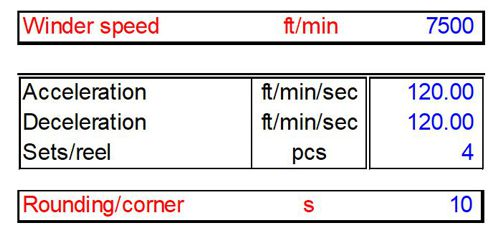Capacity calculation

The second factor that is used in the calculation is the time it takes to get from 0-speed to the constant speed and to get from constant speed back down to 0-speed - commonly referred to as acceleration and deceleration, respectively. In the capacity calculation this is how many feet/minute the speed will change in a one second time frame. Depending on paper grade this number may range, for example, from 80-200 ft/min/sec.

The last factor is the rounding time, or the time it takes to go from the accel/decel rate to constant speed. Once again, depending on the paper grade, this number will range from 5-15 seconds.

The other factor involved in the capacity calculation is measuring the winder down time. This down time comprises many factors:

• Set change
• Reel change
• Trim change
• Unwind and wind-up splices
• Splice and trim change frequency
• Web loss
• ... and a number of other factors.

Set change is the amount of time it would take for the winder to remove a finished roll and start up a new one. Valmet typically measures this from when the winder drums reach zero speed to when the drums leave zero speed. The reel change is the amount of time it takes to remove an empty spool and bring in a full spool and get the winder ready for running. The trim change is the amount of time it takes to reposition the slitters. Splices are typically measured with the zero speed reference. Web loss is calculated in a number of different ways. Some mills will weigh the paper and other mills use inches of paper (IOP) left on the reel spool. Other down times are things like web breaks and those items which are uncontrollable by the operators.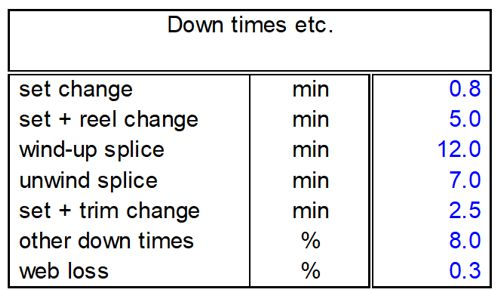Capacity calculation - down times, etc.

Down time frequency is also an item that is taken into consideration. Valmet takes this into consideration by determining how many times a certain phenomenon will take place in a 8-hour shift. Items such as trim change and splice frequency are calculated.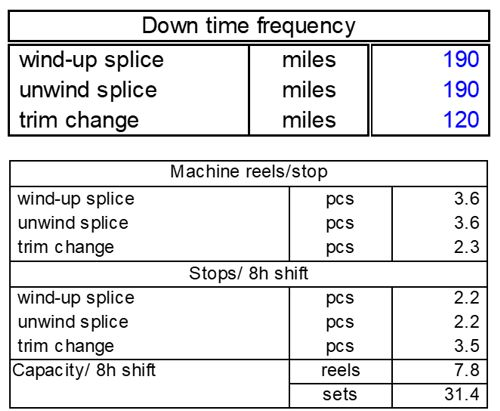Capacity calculation - down time frequency

### Interpreting capacity calculation data

When all information is entered into the calculation, four different sets of data are calculated:

• Cycle time analysis,
• Equivalent speed of paper machine,
• US-Tons/day, and
• Sets/8hr shift.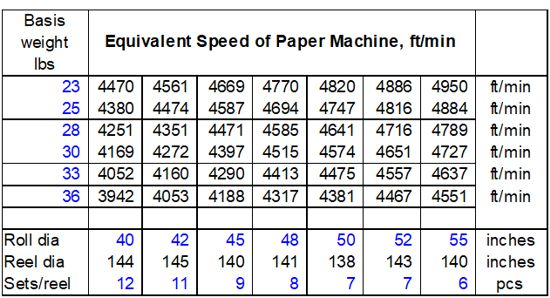Capacity calculation - interpretation

The "Equivalent Speed of Paper Machine" portion of the capacity calculation is the maximum speed that the paper machine can run if the winder is to keep up. We would look at up to 10 different basis weights and 7 different combinations on the reel (i.e. 11 sets @ 42", 7 sets @ 50").

With the same basis weight and reel combinations, the production from the winder in US-Tons/Day is calculated.

Based on the same basis weight and reel combinations, the winder production per shift (8-Hr) is calculated.

The cycle time analysis is based on all of the data produced and is used in the determination of where the capacity of the winder is being used. It is broken up into three categories:

• the amount of time it takes to run one set including set change,
• the amount of time it takes to run a complete reel, and
• the percentage of total time each function requires.

The winding total is the amount of time it takes to run a set and a reel and how much out of the total time is spent for actual running. The set change row is the amount of time it takes to complete one set change, the total time taken up for set change to expire a complete reel and how much out of the total time is spent during set change.

The other data that is produced is a break down of all other sequences that take place during the act of winding/unwinding a complete spool of paper, and the percentage of time in relation to the total time.

### Conclusion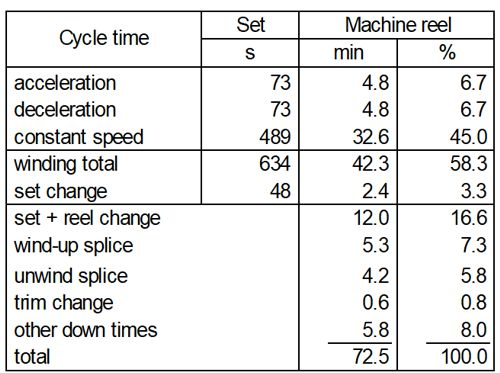Winder capacity

The gathering of the information needed to complete the capacity calculation is very important to the outcome of the capacity calculation. The calculation is only as accurate as the information that it is given. If Valmet is to do the study we will take worst case scenarios into account when calculating the capacity.